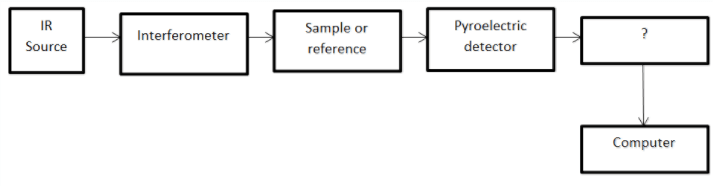# Infrared Spectrometers Questions & Answers

1. Which of the following is not true about Fourier Transform Infrared (FTIR) spectrometer?

a) It is of non-dispersive type
b) It is useful where repetitive analysis is required
c) Size has been reduced over the years
d) Size has increased over the years

Explanation: FTIR is of non-dispersive type of instruments and is used for repetitive analysis. Initially, it was bulky and cost was high and hence, it was limited to special application. Now, the size has been reduced.

2. In the most widely used beam splitter, a thin film of ________ is sandwiched between two plates of low refractive index solid. Fill the blank with the suitable option.

a) Mylar
b) Silicon carbide
c) Ferrous oxide
d) Silver chloride

Explanation: Generally, mylar is the thin film used in beam splitters. Beam splitter has to pass 50% of the radiation and reflect 50% of the radiation.

3. Which of the following is not the function of drive mechanism in Fourier Transform Infrared Spectrophotometer?

a) Movement of mirror to obtain a satisfactory interferogram
b) Acquire a good interferogram pattern
c) Allow 50% of the beam to pass
d) Keep the speed of the moving mirror constant

Explanation: Drive mechanism does the functions specified in the other options. It the function of the beam splitter to allow 50% of the beam to pass through.

4. Only pyroelectric transducer or pyroelectric crystals are used as detectors in Fourier Transform Infrared Spectrophotometer (FTIR). What is the main reason for other types of thermal detectors are not being used in FTIR spectrophotometer?

a) Less accuracy
b) Slower response
c) Less precision
d) Less sensitivity

Explanation: Other thermal detectors are not employed due to slower response of the detectors. Pyroelectric transducers or pyroelectric crystals are chosen for their high speed, accuracy, precision, sensitivity and resolution.

5. Which of the following is not the advantage of Fourier Transform Spectrometers?

a) Signal to noise ratio is high
b) Information could be obtained on all frequencies
c) Retrieval of data is possible
d) Easy to maintain

Explanation: The instrument is not easy to maintain and it is a disadvantage. The other disadvantage is that the cost is high.

6. Which of the following has to be computed to determine transmittance and absorbance at various frequencies?

a) Ratio of signal and noise
b) Ratio of sample and reference spectra
c) Sample spectra
d) Reference spectra

Explanation: The ratio of sample and reference spectra needs to be computed to determine transmittance and absorbance. First, reference interferogram is obtained and then sample is obtained.

7. Computer accepts analog signals directly.

a) True
b) False

Explanation: Computer does not accept analog signals directly. An A/D converter is required to feed the signals to the computer.

8. Which of the following is the reference that is generally used in FTIR interferometer?

a) Air
b) NaCl solution
c) Alcohol
d) Base solution

Explanation: Air is generally used as the reference. It is scanned for about 20 to 30 times and the results are stored in a computer.

9. In Michelson Interferometer, if the reflected and transmitted beams are in phase at the beam splitter, then maximum intensity will reach the detector.

a) True
b) False

Explanation: If the reflected and transmitted beams are in phase at the beam splitter then maximum intensity will reach the detector. If they are out of phase, then minimum intensity will reach the detector.

10. The diagram given below is the representation of Fourier transform interferometer. Identify the missing block in the block diagram.a) Pyroelectric crystal
b) Display
c) High speed plotter
d) A/D converter

Explanation: Computer does not accept analog signals directly. An A/D converter is required to feed the signals to the computer.

11. In Michelson’s interferometer, the frequency of the detector output can be determined by translating the _________ of movable mirror and the ___________ of monochromatic radiation.

a) Velocity, wavelength
b) Thickness, intensity
c) Length, velocity
d) Angle, intensity

Explanation: The frequency can be determined by translating the velocity of the movable mirror and the wavelength of monochromatic radiation. The reflected beam passes towards the movable mirror.

12. In Michelson’s interferometer, the __________ of the detector output will depend upon the intensity of incoming radiation.

a) Velocity
b) Frequency
c) Amplitude
d) Phase

Explanation: In Michelson’s interferometer, the amplitude of the detector output will depend upon the intensity of incoming radiation. If movable mirror is moved uniformly, the output will be a sine wave.

13. Why is the computer necessary in Fourier Transform Spectrometer?

a) To display the detector output
b) To process the detector output
c) To determine the amplitude
d) To determine the frequency

Explanation: The computer is necessary to process the output of the detector. The Fourier Transform of output is determined using software using computers.

1.